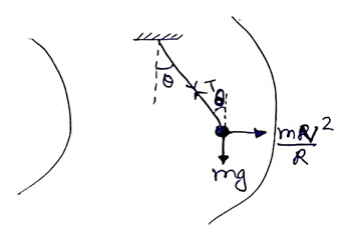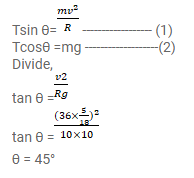# A simple pendulum is suspended from the ceiling of`
Question:

A simple pendulum is suspended from the ceiling of a car taking a turn of radius $10 \mathrm{~m}$ at a speed of $36 \mathrm{~km} / \mathrm{h}$. Find the angle made by the string of the pendulum with the vertical if this angle does not change during the turn. Take $g=10 \mathrm{~m} / \mathrm{s}^{2}$.

Solution: